# Statistical Analysis Using Fixed Effects One-Way ANOVA

QUESTION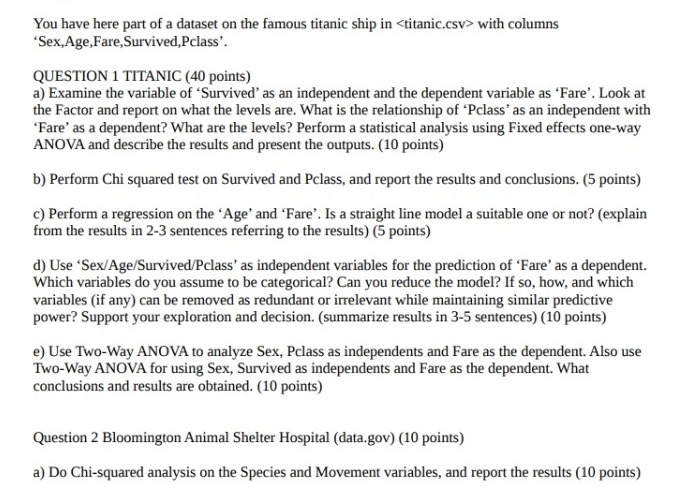# Q1. a)

The ANOVA for Survived and Fare has been added to appendix 1. The levels of Survived are 0 and 1. We can see that there is a statistically significant relationship between both the variables. Survived explains 6% of the total deviation in Fare (based on R squared value). Levene’s Test is also significant and so is Welch’s Anova.

The ANOVA for Pclass and Fare has been added to appendix 2. The levels of Pclass are 1, 2 and 3. We can see that there is a statistically significant relationship between both the variables. Pclass explains 35% of the total deviation in Fare (based on R squared value). Levene’s Test is also significant and so is Welch’s Anova.

# Q1. b)

The Chi-squared test is added to appendix 3. The test for equal means is statistically significant. Thus we reject the null hypothesis that the means are equal. As dichotomous variables are involved, thus the Phi coefficient and Cramer’s V also should be significant which they are.

# Q1. c)

The results of regression are added to appendix 4. The results show that Age is a significant factor of Fare at 95% level of confidence. The variable however explains less than 1% of the total deviation in fare. Based on the plot between predicted values and Fare, a straight line model might not be an ideal model for Fare.

# Q1. d)

The regression analysis is added to appendix 5. Survived, Pclass and Sex are assumed to be categorical variable. The model is able to predict approximately 39% of deviation in fare. We can see that survived is not a significant factor at 95% level of confidence and it can be reduced from the model. Removing the variable should not reduce the power of the model much.

# Q1. e)

Two ways Anova for Sex, Pclass and Fare is added to appendix 6. We can see that there is a statistically significant relationship between the variables. The variables explain 36% of the total deviation in Fare (based on R squared value). Turkey-Kramer results are statistically significant which makes the results of Anova valid.

Two ways Anova for Sex, Survived and Fare is added to appendix 6. We can see that there is a statistically significant relationship between the variables. Individually Sex is not a significant in the analysis at 95% level of confidence. The variables explain 6.8% of the total deviation in Fare (based on R squared value). Turkey-Kramer results are statistically significant which makes the results of Anova valid.

# Q2. a)

The chi-square results are added in appendix 8. Both cross and individual test have been performed. The test for equality of means is statistically significant and hence the means are not equal. The individual test for both the variables is also significant which means that the frequency distribution in each variable is also not similar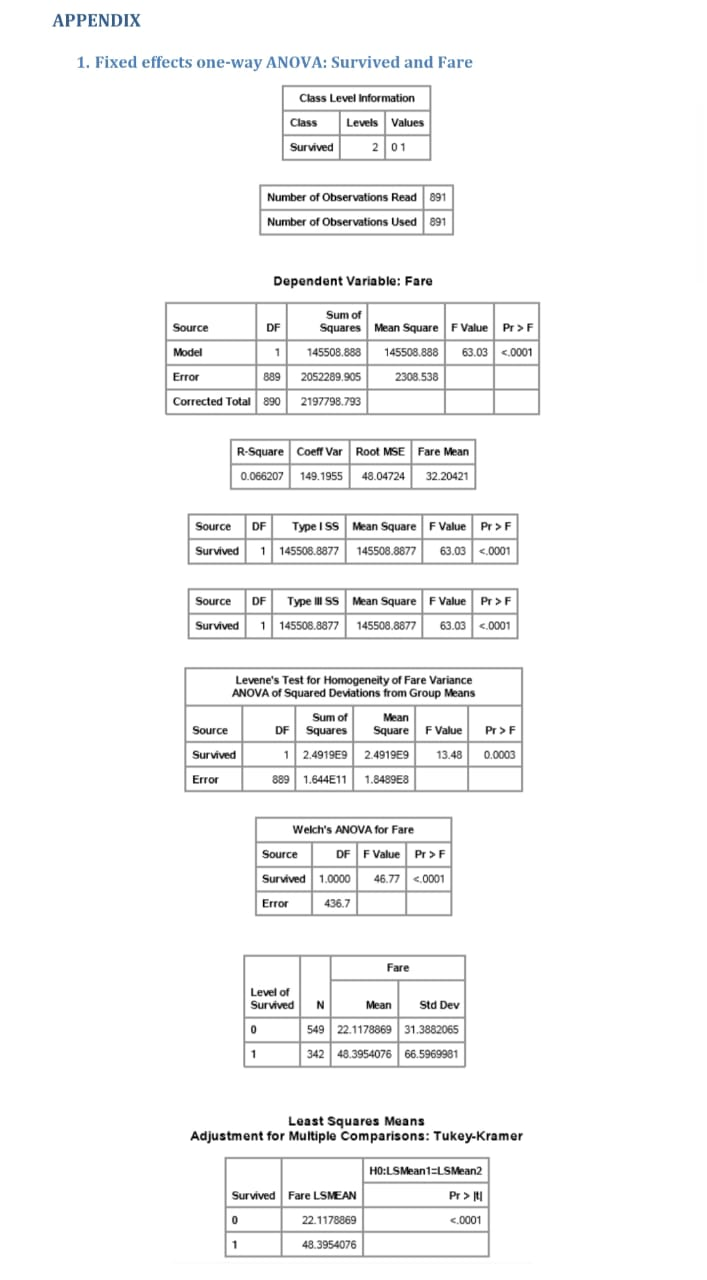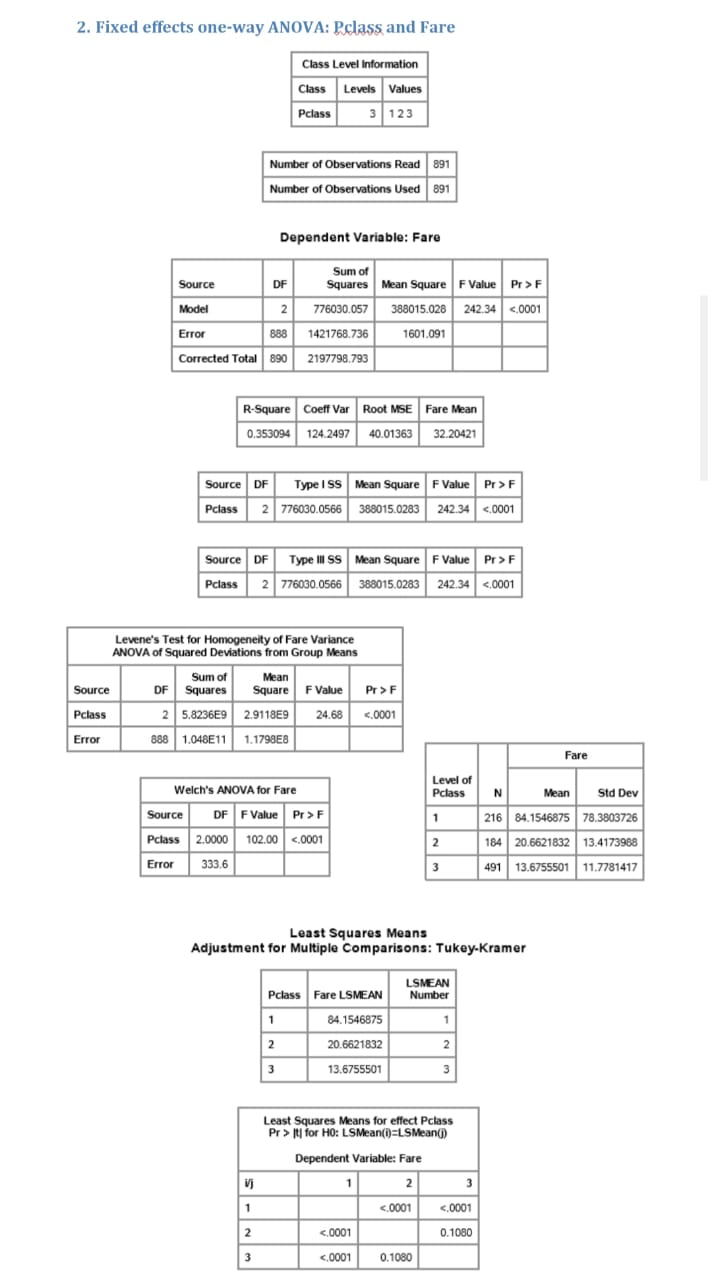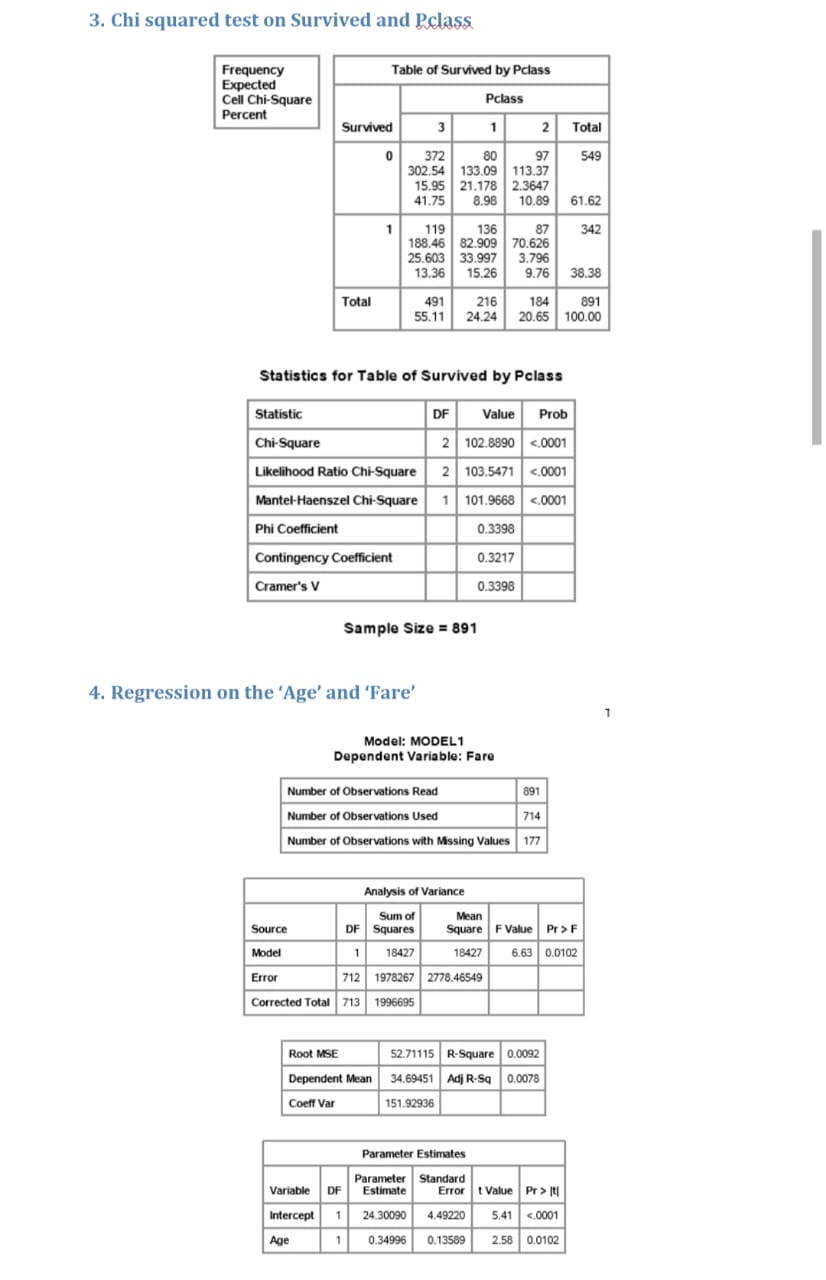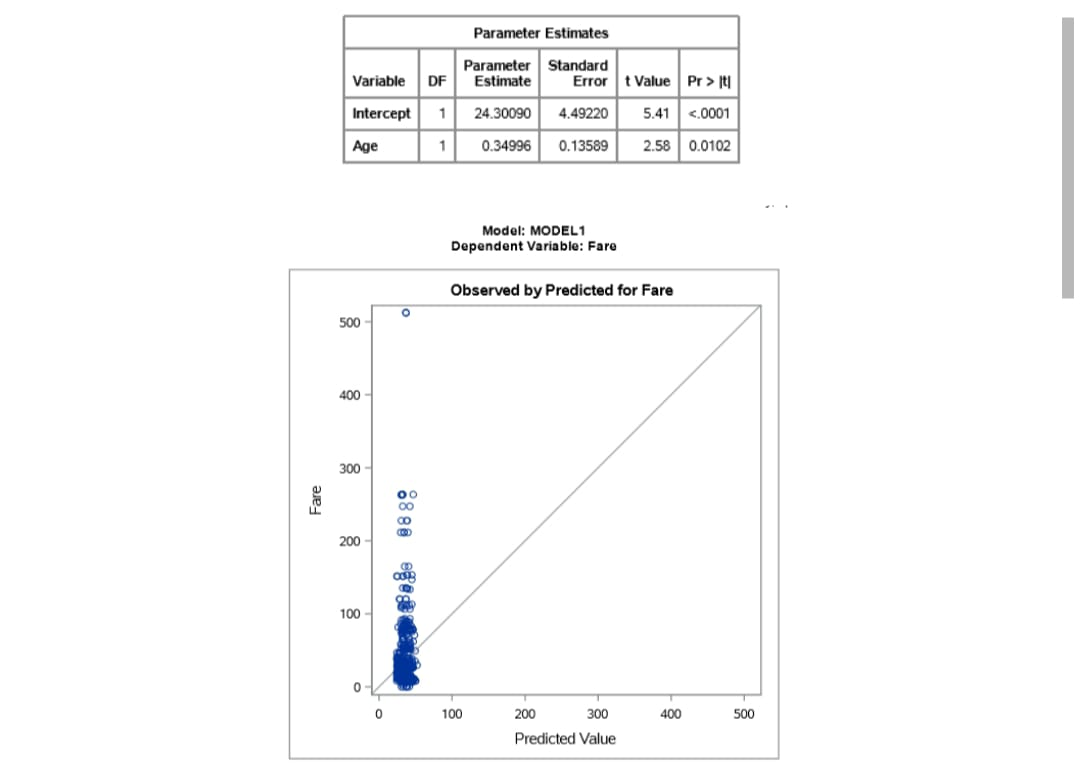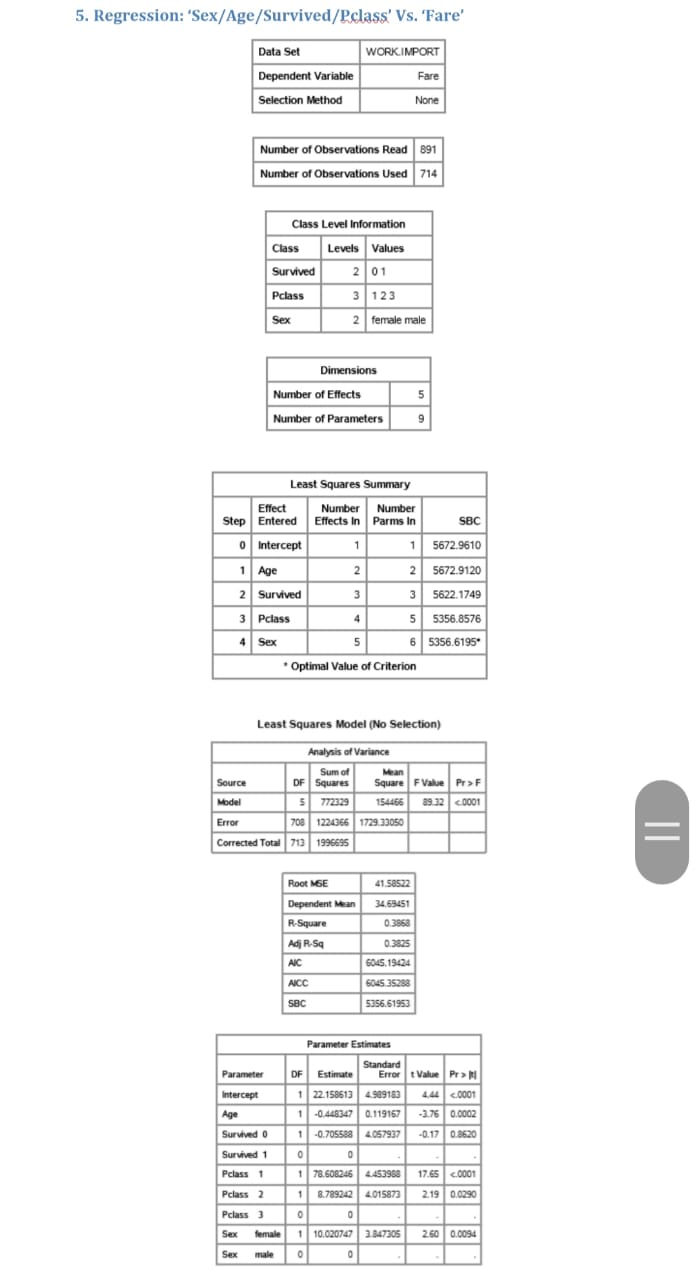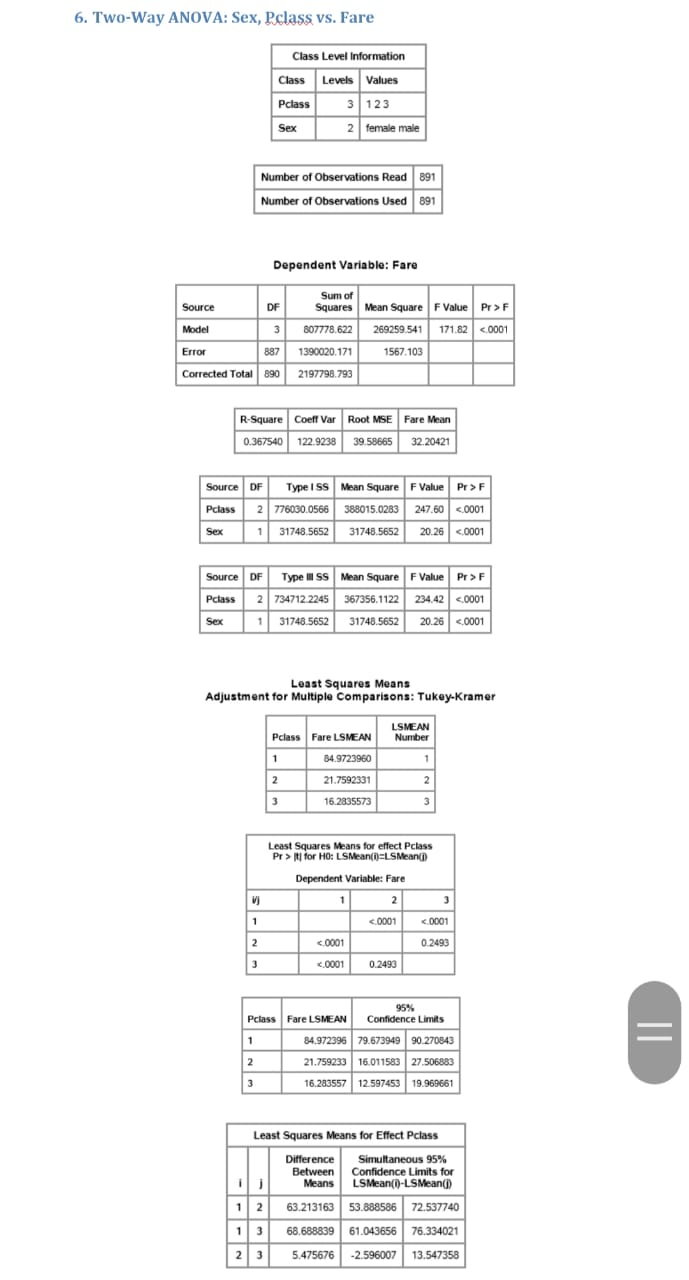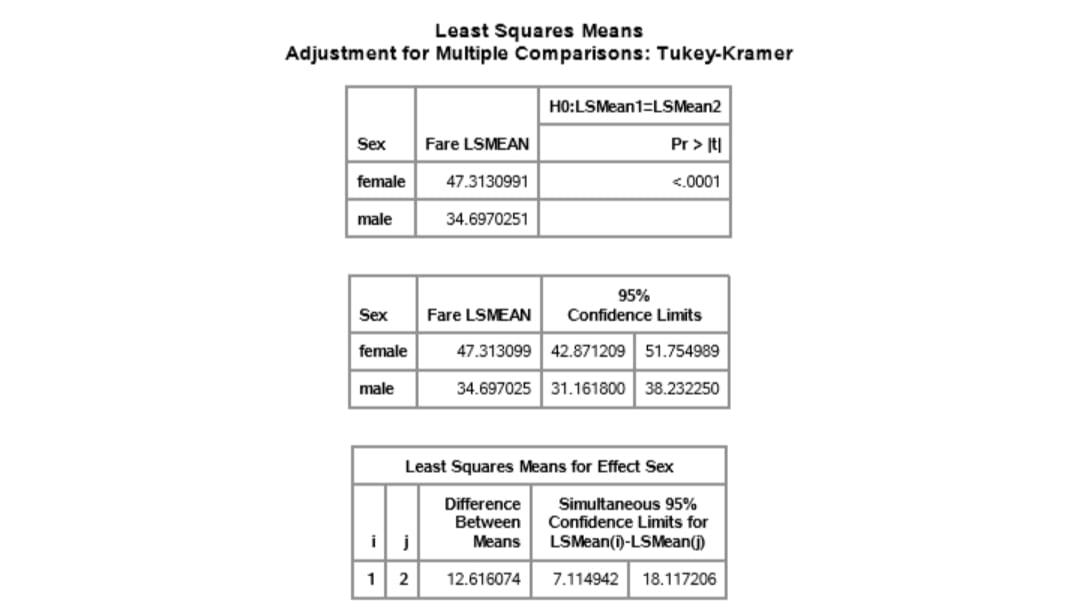Looking for Statistics Assignment Help. Whatsapp us at +16469488918 or chat with our chat representative showing on lower right corner or order from here. You can also take help from our Live Assignment helper for any exam or live assignment related assistance.